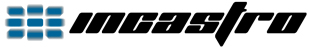Back

Android - OpenGL ES 1 - Tutorial 7 - Blending Colors

In the following screenshot, the two squares have a common area and their colors are mixed:To achieve this, just enable the operation and define the mixing mode. We do this in the "onSurfaceCreated()" method:
```// Enable blending.
gl.glEnable(GL10.GL_BLEND);
// Blending function.
gl.glBlendFunc(GL10.GL_ONE, GL10.GL_ONE);```
The general formula is:

output = (source factor * source fragment) + (destination factor * destination fragment)

where:
- source factor is the first parameter of the function (GL_ONE)
- source fragment is the green square
- destination factor is the second parameter of the function (GL_ONE)
- destination fragment is the red square

In our case, the RGBA components of the green square are multiplied by 1 and added to the RGBA components of the red square multiplied by 1.

To obtain the transparency effect when two textures are overlapped:we have to set the function as follows:
```// Blending function.
gl.glBlendFunc(GL10.GL_ONE, GL10.GL_ONE_MINUS_SRC_ALPHA);```
OpenGL ES 1 Tutorial 7
OpenGL ES 1 Tutorial 7bBack